# Linearized Reduced Drift-MHD Equations

Let us now take the tearing mode perturbation into account. In accordance with Equations (5.22)–(5.25), and (5.30)–(5.31), we can write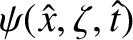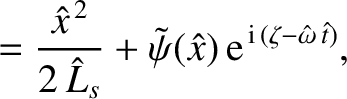(5.33)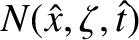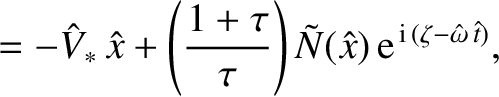(5.34)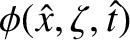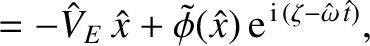(5.35)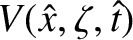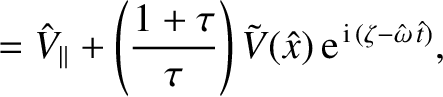(5.36)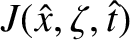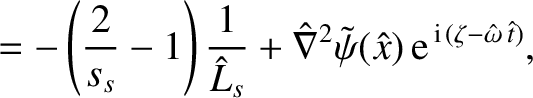(5.37)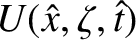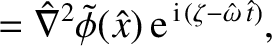(5.38)

where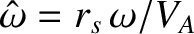, and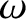is the rotation frequency of the tearing perturbation in the laboratory frame. Here,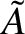denotes a perturbed quantity. Substituting Equations (5.33)–(5.38) into the reduced drift-MHD model, (5.8)–(5.13), and only retaining terms that are first order in perturbed quantities, we obtain the following set of linear equations: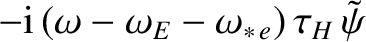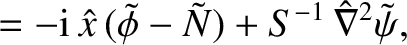(5.39)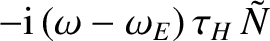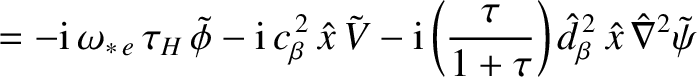(5.40)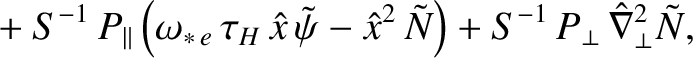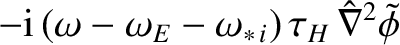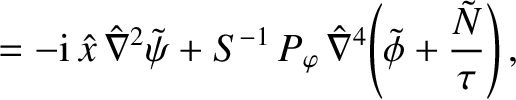(5.41)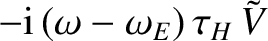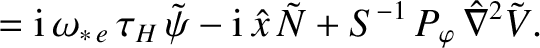(5.42)

Here,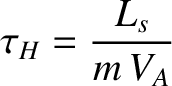(5.43)

is the hydromagnetic time,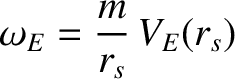(5.44)

the E-cross-B frequency,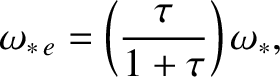(5.45)

the electron diamagnetic frequency,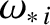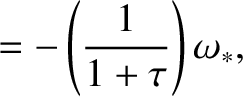(5.46)

the ion diamagnetic frequency,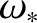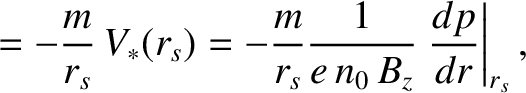(5.47)

the (total) diamagnetic frequency,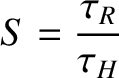(5.48)

the Lundquist number [note that this is a slightly different definition to that given in Equation (1.84)],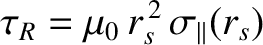(5.49)

the resistive diffusion time [note that this is a slightly different definition to that given in Equation (1.83)],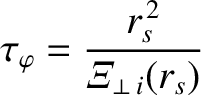(5.50)

the toroidal momentum confinement time,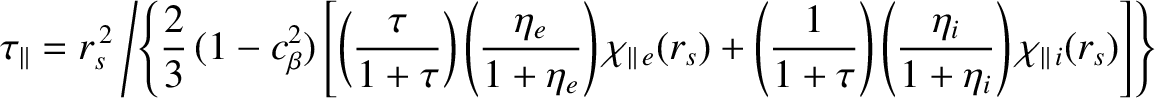(5.51)

the effective parallel energy equilibration time, and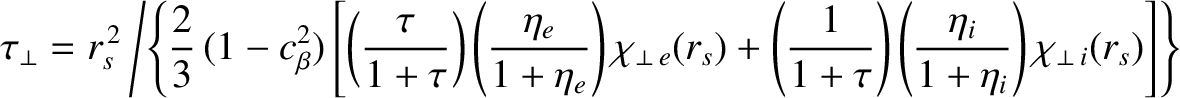(5.52)

the effective energy confinement time. Furthermore,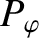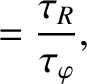(5.53)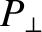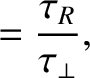(5.54)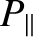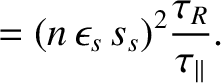(5.55)